Gauge your potential with Aakash BYJU'S JEE Main'23 Mock Test Gauge your potential with Aakash BYJU'S JEE Main'23 Mock Test

# JEE Main 2021 March 18 – Shift 2 Physics Question Paper with Solutions

JEE Main 2021 March 18th shift 2 Physics question paper with solutions have been provided on this page. These solutions have been prepared by subject matter experts at BYJU’S. Revising these questions and solutions will help students to get a clearer idea of the, question types, marking scheme and pattern of the JEE Main exam. The question paper with solutions can also be downloaded in a PDF format. Practicing these solutions will help students to boost their confidence and score more marks in the upcoming exams.

### JEE Main 2021 March 18th Shift 2 Physics Question Paper

Section - A

Question 1. The decay of a proton to neutron is:

1. a. Not possible as proton mass is less than the neutron mass
2. b. Always possible as it is associated only with β+ decay
3. c. Possible only inside the nucleus
4. d. Not possible but neutron to proton conversion is possible

Solution:

Positron emission or Beta plus decay is a subtype of radioactive decay called Beta decay, in which a proton inside a radionuclide nucleus is converted into a neutron while releasing a positron and an electron neutrino. So, the decay of a proton to a neutron is possible only inside the nucleus.

Question 2: An object of mass m1 collides with another object of mass m2, which is at rest. After the collision, the objects move at equal speeds in opposite directions. The ratio of the masses m2: m1 is:

1. a. 2: 1
2. b. 1: 1
3. c. 1: 2
4. d. 3: 1

Solution: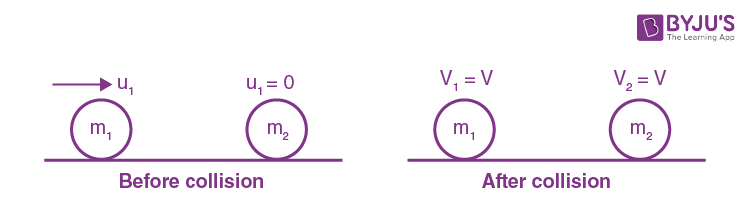From the conservation of linear momentum;

Pi = Pf

⇒m1u1+m2(0)=m1(-v)+m2v

⇒m1u1=v(m2-m1) …(i)

Since,

Since, e=1= (Vsep/Vapp) =v-(-v)/u1=2v/u1

⇒ u1=2v …(ii)

from (i) & (ii)

m1(2v)=v(m2-m1)

⇒2m1=m2 - m1

⇒3m1=m2

⇒ m2/m1=3/1

Question 3. A plane electromagnetic wave propagating along y-direction can have the following pair of electric field (

$$\vec{E}$$
)and magnetic field (
$$\vec{B}$$
) components.

1. a. Ex, Bz or Ez, Bx
2. b. Ey, Bx or Ex, By
3. c. Ex, By or Ey, Bx
4. d. Ey, By or Ez, Bz

Solution:Therefore,

$$\hat{E}\times \hat{B}=\hat{C}$$
for electromagnetic waves

Therefore,

$$\hat{E}\times \hat{B}$$
should point in the

direction of propagation of wave (y-direction here)

Therefore, the possible combinations are

(Ex , Bz) or (Ez , Bx)

Question 4: A solid cylinder of mass m is wrapped with an inextensible light string and is placed on a rough inclined plane as shown in the figure. The frictional force acting between the cylinder and the inclined plane is :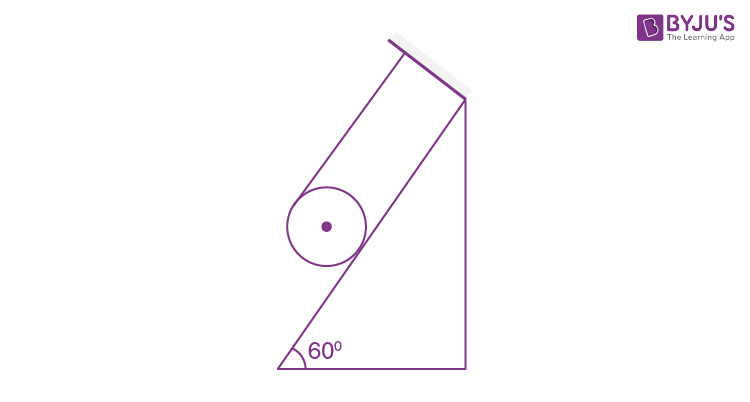[The coefficient of static friction, μs, is 0.4]

1. a. 72mg
2. b. 0
3. c. mg/5
4. d. 5 mg

Solution: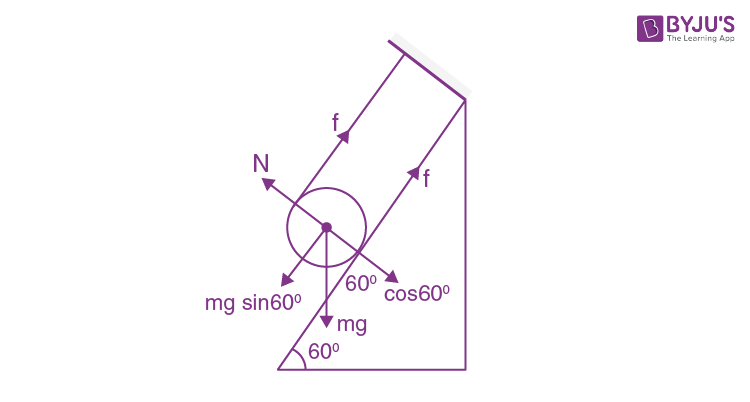Let's assume equilibrium condition of cylinder

Therefore, T+f= mgsin60° ….(i)

& TR – fR = 0 ….(ii)

from (i) & (ii)

T=freq=mgsin60°/2

But limiting friction < required friction.

Therefore, μmgcos60° <mgsin60°/2

Therefore, Cylinder won't be in equilibrium

f will be kinetic

Since the coefficient of kinetic friction is not mentioned, we assume

coefficient of kinetic friction = coefficient of static friction.

& f = μK N

kmgcos60°

=0.4×mg×(½)=mg/5

Question 5. An ideal gas in a cylinder is separated by a piston in such a way that the entropy of one part is S1 and that of the other part is S2. Given that S1 > S2. If the piston is removed then the total entropy of the system will be:

1. a. S1 + S2
2. b. S1 - S2
3. c. S1 × S2
4. d. S1/S2

Solution: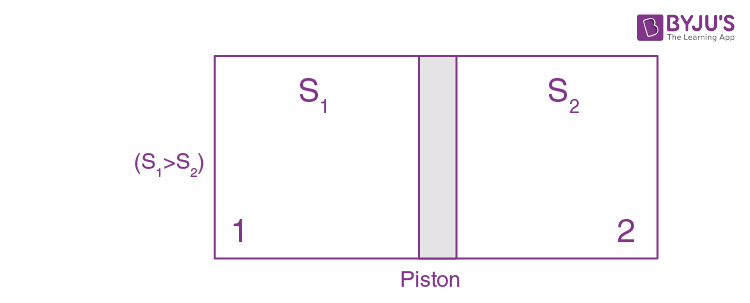for gas 1 , S1= (f/2n1R)

for gas 2, S2=(f/2n2R} identical gas, so f will be the same.

after removal of piston,

s= f/2(n1+n2)R=S1+S2

Question 6. The time taken for the magnetic energy to reach 25% of its maximum value, when a solenoid of resistance R, inductance L is connected to a battery, is :

1. a. (L/R)ln2
2. b. (L/R)ln10
3. c. Infinite
4. d. (L/R)ln5

Solution:

Magnetic energy, U=(½)LI2, when the current in the circuit is I.

→ When the circuit has maximum current,

Maximum value of Magnetic energy, U0=(½)LI02

Given : U = 25% of U0.

$$\Rightarrow \frac{1}{2}LI^{2}=\frac{1}{4}\times \frac{1}{2}LI_{0}^{2}$$

$$I^{2}=\frac{I_{0}^{2}}{4}\Rightarrow I=\frac{I_{0}}{2}$$

Therefore, I = I0(1 - e-t/τ)

$$\Rightarrow \frac{I_{0}}{2}=I_{0}(1-e^{-t/\tau })$$

$$\frac{1}{2}=-e^{-t/\tau }$$

$$\Rightarrow e^{t/\tau }=2$$

$$\Rightarrow t=\tau ln2$$

$$\Rightarrow t=\frac{L}{R} ln2$$

Question 7. For an adiabatic expansion of an ideal gas, the fractional change in its pressure is equal to (where λ is the ratio of specific heats) :

1. a.
$$-\gamma \frac{dV}{V}$$
2. b. dV/V
3. c.
$$-\frac{1}{\gamma } \frac{dV}{V}$$
4. d.
$$-\gamma \frac{V}{dV}$$

Solution:

PVγ=const.

⇒lnP+γlnV=const.

⇒ differentiating both sides;

(dP/P) + γ(dV/V)=0

⇒dP/P=-γ(dV/V)

Question 8. The correct relation between α (ratio of collector current to emitter current) and β (ratio of collector current to base current) of a transistor is :

1. a.
$$\alpha =\frac{\beta }{1+\beta }$$
2. b.
$$\alpha =\frac{\beta }{1-\alpha }$$
3. c.
$$\beta =\frac{1 }{1-\alpha }$$
4. d.
$$\beta =\frac{\alpha}{1+\alpha }$$

Solution:

$$\alpha =\frac{I_{C}}{I_{E}}$$

$$\beta =\frac{I_{C}}{I_{B}}$$

Since, IE = IB + IC

Therefore,

$$\frac{I_{E}}{I_{C}}=\frac{I_{B}}{I_{C}}+\frac{I_{C}}{I_{C}}$$

$$\Rightarrow \frac{1}{\alpha }=\frac{1}{\beta }+1=\frac{1+\beta }{\beta }$$

$$\Rightarrow \alpha =\frac{\beta }{1+\beta }$$

Question 9. In a series LCR circuit, the inductive reactance (XL) is 10 Ω and the capacitive reactance (XC) is 4 Ω. The resistance (R) in the circuit is 6 Ω. Find the power factor of the circuit.

1. a.
$$\frac{1}{\sqrt{2}}$$
2. b.
$$\frac{\sqrt{3}}{2}$$
3. c. ½
4. d.
$$\frac{1}{2\sqrt{2}}$$

Solution:

Given : XL = 10 Ω

XC = 4 Ω

R = 6 Ω

Therefore,

$$Power factor = cos \theta = R/Z$$

$$= \frac{R}{\sqrt{R^{2}+(X_{L}-X_{C})^{2}}}$$

$$= \frac{6}{\sqrt{6^{2}+(10-4)^{2}}}$$

$$= \frac{6}{6\sqrt{2}}$$

$$= \frac{1}{\sqrt{2}}$$

Question 10. A proton and an α-particle, having kinetic energies Kp and Kα respectively, enter into a magnetic field at right angles. The ratio of the radii of the trajectory of proton to that α-particle is 2: 1. The ratio of KP: Kα is :

1. a. 1 : 8
2. b. 1 : 4
3. c. 8 : 1
4. d. 4 : 1

Solution:

Since,

$$r = \frac{mv}{qB}=\frac{p}{qB}$$

And

$$\frac{m_{a}}{m_{p}}=\frac{4}{1}$$

Since,

$$\frac{r_{p}}{r_{\alpha }}=\frac{P_{p}}{P_{\alpha }}.\frac{q_{\alpha }}{q_{p}}=\frac{2}{1}$$

$$\Rightarrow \frac{P_{p}}{P_{\alpha }}=\frac{2q_{p}}{q_{\alpha }}=2\left ( \frac{1}{2} \right )=1$$

Now,

$$\frac{K_{p}}{K_{\alpha }}=\left ( \frac{P_{p}}{P_{\alpha }} \right )^{2}.\frac{m_{\alpha }}{m_{p}}=1\times 4=4$$

Question 11. The function of time representing a simple harmonic motion with a period of π/ω is :

1. a. cos(ωt) + cos(2ωt) + cos(3ωt)
2. b. 3cos(π/4-2ωt)
3. c. sin2(ωt)
4. d. sin(ωt) + cos(ωt)

Solution:

For expression, 3cos(π/4-2ωt)

Angular frequency =2ω

Time period, T = 2π(2ω)= π/ω

Question 12. Consider a uniform wire of mass M and length L. It is bent into a semicircle. Its moment of inertia about a line perpendicular to the plane of the wire passing through the centre is :

1. a.
$$\frac{1}{2}\frac{ML^{2}}{\pi ^{2}}$$
2. b.
$$\frac{2}{5}\frac{ML^{2}}{\pi ^{2}}$$
3. c.
$$\frac{1}{4}\frac{ML^{2}}{\pi ^{2}}$$
4. d.
$$\frac{ML^{2}}{\pi ^{2}}$$

Solution: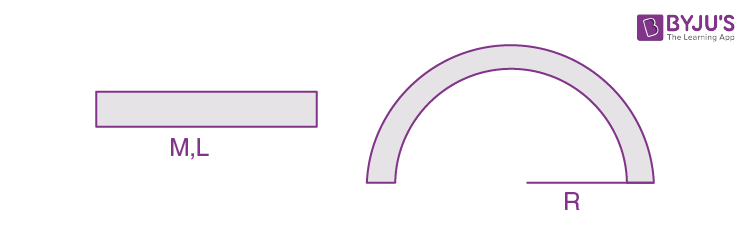∵ L=πR

⇒R=L/π

& moment of inertia = MR2 = M(L/π)2=ML22

Question 13. The angular momentum of a planet of mass M moving around the sun in an elliptical orbit is

$$\vec{L}$$
. The magnitude of the areal velocity of the planet is :

1. a. L/M
2. b. 2L/M
3. c. L/2M
4. d. 4L/M

Solution:

Theoretical concept.

∴ Areal velocity, dA/dt=L/2M

→ L = Angular momentum of planet

M = Mass of planet.

Question 14. The velocity – displacement graph of a particle is shown in the figure.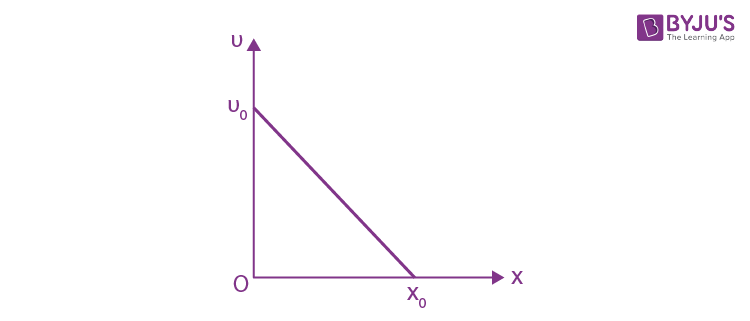The acceleration – displacement graph of the same particle is represented by: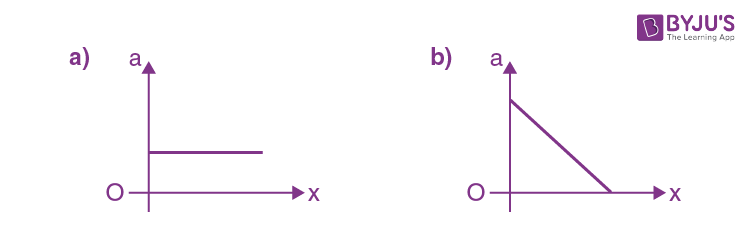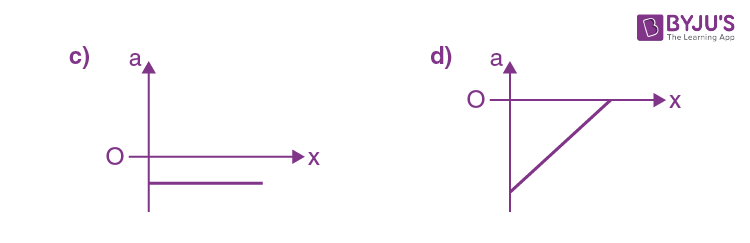Solution: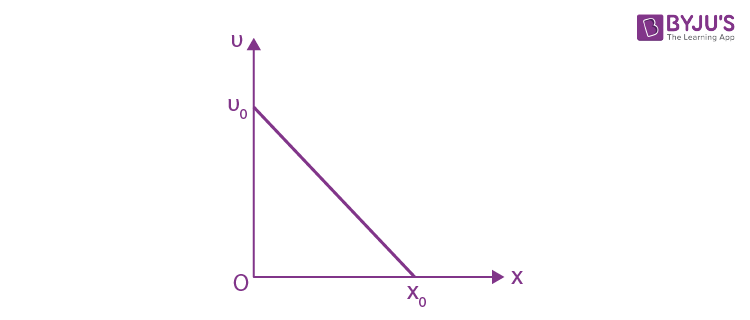Slope of given graph,

$$m= \frac{-v_{0}}{x_{0}}$$

Therefore,

$$v = \left (\frac{-v_{0}}{x_{0}}\right )x +v_{0}$$

And ,

$$a = \frac{vdv}{dx}$$

$$a = \left [ (\frac{-v_{0}}{x_{0}})x + v_{0}\right ].\frac{d}{dx}\left [ \left (\frac{-v_{0}}{x_{0}}x + v_{0}\right ) \right ]$$

$$a = \left [ (\frac{-v_{0}}{x_{0}})x + v_{0}\right ].\left [ \left (\frac{-v_{0}}{x_{0}} + 0\right ) \right ]$$

$$= \frac{v_{0}^{2}}{x_{0}^{2}}x - \frac{v_{0}^{2}}{x_{0}}$$

Again comparing with standard equation of straight line (y = mx + c)

Here, m = +ve & c = -ve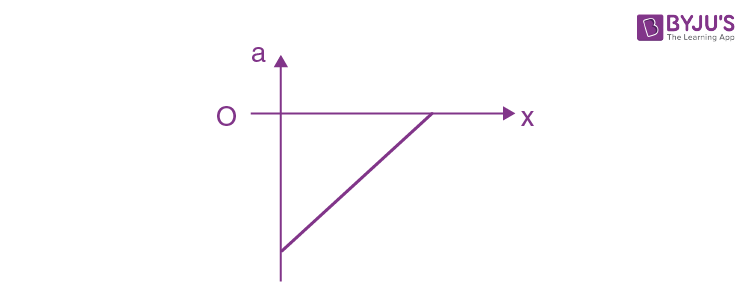∴ only graph (4) is possible.

Question 15. Three rays of light, namely red (R), green (G) and blue (B) are incident on the face PQ of a right angled prism PQR as shown in the figure.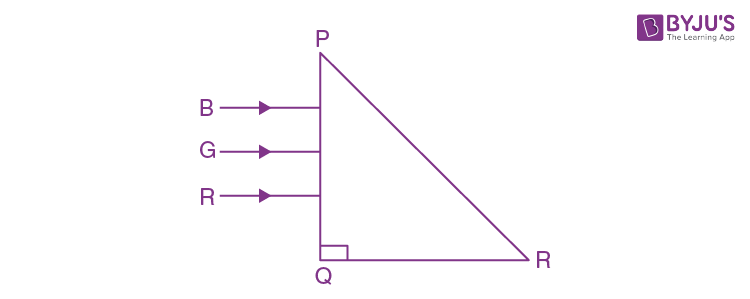The refractive indices of the material of the prism for red, green and blue wavelengths are 1.27, 1.42 and 1.49 respectively. The colour of the ray(s) emerging out of the face PR is:

1. a. Blue
2. b. Green
3. c. Red
4. d. Blue and green

Solution: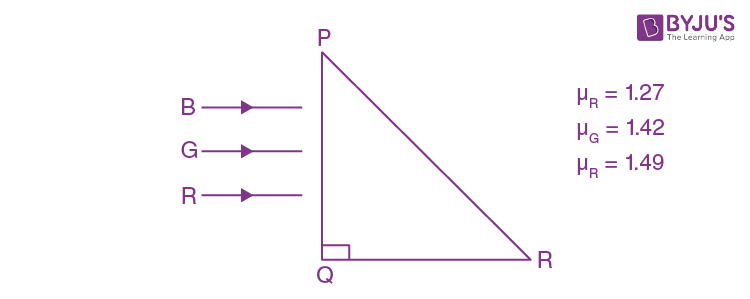For TIR, at face PR, i = 45°

& i > C ⇒ 45°>C

sin c = 1/μ

⇒ c=sin-1(1/μ)

μ > 2

μ > 1.414

∴ μG and μB are more than μ.

∴ only red will come out.

Question 16. Consider a sample of oxygen behaving like an ideal gas. At 300 K, the ratio of root mean square (rms) velocity to the average velocity of gas molecule would be : (Molecular weight of oxygen is 32 g/mol; R=8.3 JK-1 mol-1)

1. a.
$$\sqrt{\frac{3}{3}}$$
2. b.
$$\sqrt{\frac{8}{3}}$$
3. c.
$$\sqrt{\frac{3\pi }{8}}$$
4. d.
$$\sqrt{\frac{8\pi }{3}}$$

Solution:

$$V_{rms}=\sqrt{\frac{3RT}{M}}$$

And

$$V_{avg}=\sqrt{\frac{8RT}{\pi M}}$$

Therefore,

$$\frac{V_{rms}}{V_{avg}}=\sqrt{\frac{3\pi }{8}}$$

Question 17. A particle of mass m moves in a circular orbit under the central potential field, U(r)= -C/r, where C is a positive constant. The correct radius – velocity graph of the particle's motion is :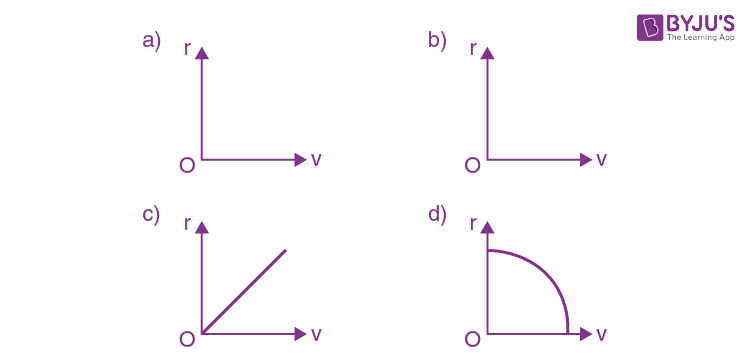Solution:Given potential field. U(r)=-C/r

∵F=-dU/dr=C/r2

& FC = mv2/r

∴ mv2/r=C/r2

⇒r=C/mv2

⇒r α1/v2

∴ The graph between r & v will be hyperbolic.

Question 18. Which of the following statements are correct ?

(A) Electric monopoles do not exist whereas magnetic monopoles exist.

(B) Magnetic field lines due to a solenoid at its ends and outside cannot be completely straight and confined.

(C) Magnetic field lines are completely confined within a toroid.

(D) Magnetic field lines inside a bar magnet are not parallel.

(E) χ = —1 is the condition for a perfect diamagnetic material, where χ is its magnetic susceptibility.

Choose the correct answer from the options given below :

1. a. (B) and (C) only
2. b. (B) and (D) only
3. c. (C) and (E) only
4. d. (A) and (B) only

Solution:

(A) Electric monopoles exist while magnetic monopoles do not exist.

(B) Magnetic field lines at the ends and outside of solenoid cannot be confined.

(C) Magnetic field lines are confined within a toroid.

(D) Magnetic field lines inside a bar magnet are parallel.

(E) For perfectly diamagnetic material χ = -1

Therefore, (C) & (E) are correct.

Question 19. The speed of electrons in a scanning electron microscope is 1×107 ms-1. If the protons having the same speed are used instead of electrons, then the resolving power of scanning proton microscope will be changed by a factor of:

1. a.
$$\frac{1}{\sqrt{1837}}$$
2. b.
$$\sqrt{1837}$$
3. c. 1837
4. d. 1/1837

Solution:

RP ∝ 1/λ

And λ ∝ 1/m

⇒ RP ∝ m

Therefore,

$$\frac{RP_{pr}}{RP_{el}}=\frac{m_{pr}}{m_{el}} = 1837$$

Question 20. If the angular velocity of earth's spin is increased such that the bodies at the equator start floating, the duration of the day would be approximately :

[Take g = 10 ms-2, the radius of earth, R = 6400×103 m, Take π = 3.14]

1. a. 60 minutes
2. b. does not change
3. c. 84 minutes
4. d. 1200 minutes

Solution:

At the equator, the body starts floating i.e. condition of weightlessness.

∵gequ=g- Rω2=0

$$\omega =\sqrt{\frac{g}{R}}$$

∴ Time period, T = 2π/ω

$$=2\pi \sqrt{\frac{R}{g}}$$

=

$$= 2\times 3.14\sqrt{\frac{6.4\times 10^{6}}{10}}$$

≃84 minutes

SECTION-B

Question 21. Two wires of same length and thickness having specific resistances 6 Ω cm and 3 Ω cm respectively are connected in parallel. The effective resistivity is ρ Ω cm. The value of ρ, to the nearest integer, is _________.

Solution:In parallel,

$$R_{eq}=\frac{R_{1}R_{2}}{R_{1}+R_{2}}$$

$$\frac{\rho l}{2A}=\frac{\rho _{1}\frac{l}{A}\times \rho _{2}\frac{l}{A} }{\rho _{1}\frac{l}{A}+\rho _{2}\frac{l}{A}}$$

$$\Rightarrow \frac{\rho }{2}=\frac{6\times 3}{6+3}=2$$

⇒ ρ = 2 x 2 = 4

Question 22. A ball of mass 4 kg, moving with a velocity of 10 ms-1, collides with a spring of length 8 m and force constant 100 Nm-1. The length of the compressed spring is x m. The value of x, to the nearest integer, is _______

Solution:

If spring is compressed by y

Applying energy conservation principle,

$$\frac{1}{2}mv^{2}=\frac{1}{2}ky^{2}$$

$$y=\sqrt{\frac{m}{k}}.v=\sqrt{\frac{4}{100}}\times 10= 2 m$$

∴ final length of spring = 8 – 2 = 6 m

Question 23. Consider a 72 cm long wire AB as shown in the figure. The galvanometer jockey is placed at P on AB at a distance x cm from A. The galvanometer shows zero deflection.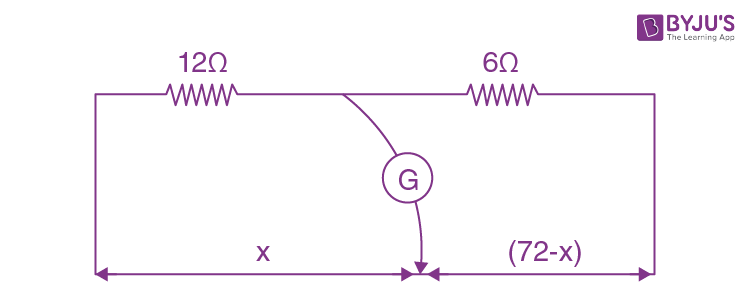The value of x, to the nearest integer, is ___________

Solution:At balanced condition

$$\frac{x}{12}=\frac{72-x}{6}$$

⇒x=2(72-x)

⇒3x=144

⇒x=144/3= 48 cm

Question 24. Consider a water tank as shown in the figure. It's cross-sectional area is 0.4 m2. The tank has an opening B near the bottom whose cross-sectional area is 1 cm2. A load of 24 kg is applied on the water at the top when the height of the water level is 40 cm above the bottom, the velocity of water coming out the opening B is v ms-1. The value of v, to the nearest integer, is …………

[Take value of g to be 10 ms-2]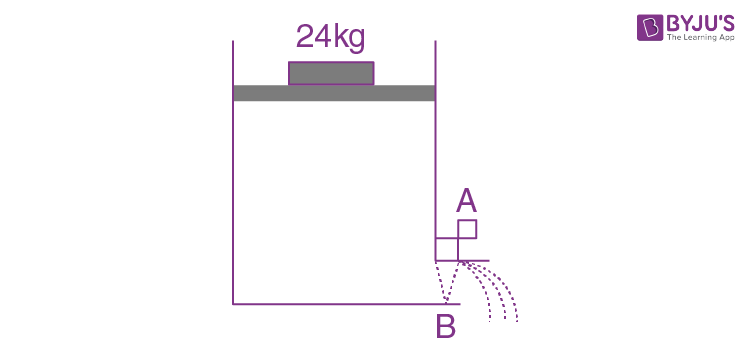Solution: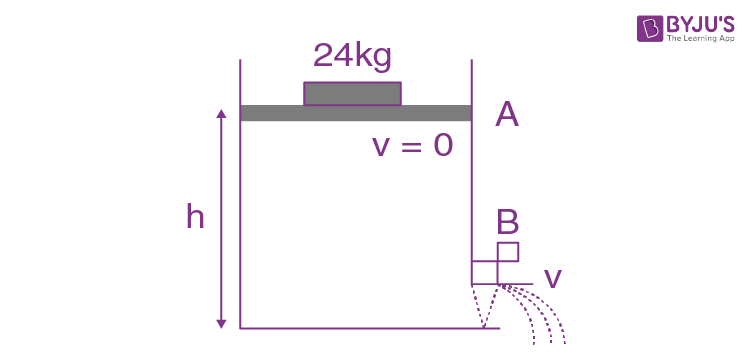On applying Bernoulli's theorem at points A & B

$$P_{0}+\frac{mg}{A}+\rho gh+\frac{1}{2}\rho V^{2}= P_{0} + \frac{1}{2}\rho v^{2}$$

Therefore, V = 0 (at A)

Since,

$$\frac{mg}{A}+\rho gh = \frac{1}{2}\rho v^{2}$$

$$\Rightarrow \frac{24\times 10}{0.4} + 10^{3}\times 10\times 0.4 = \frac{1}{2}\times 10^{3}v^{2}$$

⇒v≃3 m/s

Question 25. The typical output characteristics curve for a transistor working in the common emitter configuration is shown in the figure.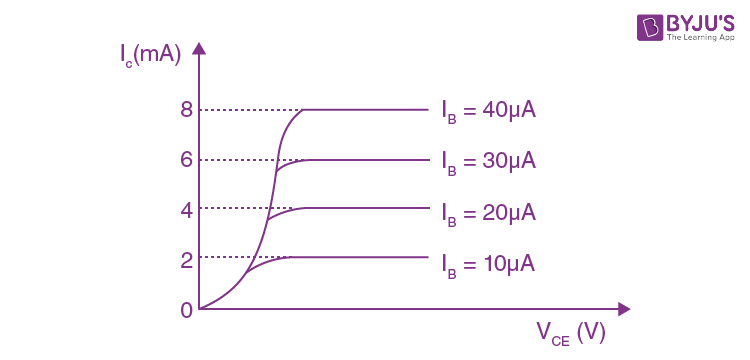The estimated current gain from the figure is …………..

Solution:

For common emitter configuration

$$\beta =\frac{\Delta I_{c}}{\Delta I_{B}}= \frac{(4-2)mA}{(20-10)\mu A}= \frac{2\times 10^{-3}}{10\times 10^{-6}}= 200$$

Question 26. The radius of a sphere is measured to be (7.50 ± 0.85) cm. Suppose the percentage error in its volume is x. The value of x, to the nearest integer x, is ………………

Solution:

$$V = \frac{4}{3}\pi r^{3}$$

$$\frac{\Delta V }{V}\times 100 = 3\frac{\Delta r}{r}\times 100$$

$$= 3\left ( \frac{0.85}{7.5} \right )\times 100$$

$$= \left ( \frac{2.55}{7.5} \right )\times 100$$

=34%

Question 27. The projectile motion of a particle of mass 5 g is shown in the figure.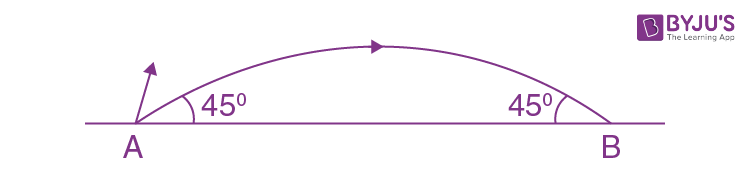The initial velocity of the particle is 5√2 ms-1 and the air resistance is assumed to be negligible. The magnitude of the change in momentum between the points A and B is X × 102 kgms-1. The value of X, to the nearest integer, is …………………..

Solution: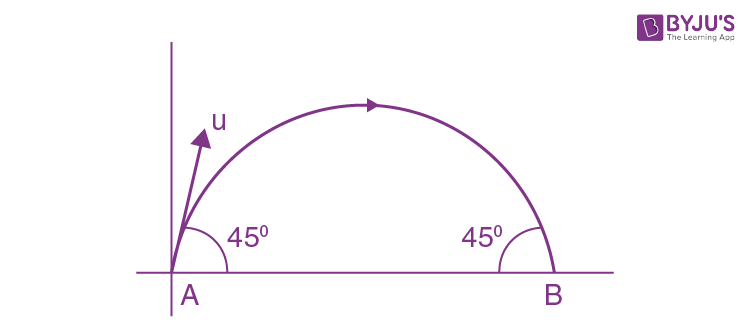$$\vec{\Delta P} = \vec{P_{B}}-\vec{P_{A}}$$

$$= m(5\sqrt{2}cos 45^{0}\hat{i}-5\sqrt{2}sin45^{0}\hat{j})$$

$$= - m(5\sqrt{2}cos 45^{0}\hat{i}+5\sqrt{2}sin45^{0}\hat{j})$$

$$= - 2m \times 5\sqrt{2}\times \frac{1}{\sqrt{2}}\hat{j}$$

= –10×5×10-3 kg m/s

$$\left | \vec{P} \right |= 5 \times 10^{-2} kg m/s$$

∴ X=5

Question 28. An infinite number of point charges, each carrying 1 μC charge, are placed along the y-axis at y = 1 m, 2 m, 4 m, 8 m ………………….. The total force on a 1 C point charge, placed at the origin, is X × 103 N. The value of X, to the nearest integer, is ……… [Take 1/4𝜋∈0 = 9×109 Nm2/C2]

Solution:$$F_{total}= \frac{kq_{1}q_{2}}{r_{1}^{2}} + \frac{kq_{1}q_{2}}{r_{2}^{2}} + \frac{kq_{1}q_{2}}{r_{3}^{2}}+ -----$$

$$= 9\times 10^{9}\times 10^{-6}\left [ 1+\left (\frac{1}{2} \right ) + \left ( \frac{1}{2^{2}} \right )^{2} + \left ( \frac{1}{2^{3}} \right )^{2}+ -----\left ( \frac{1}{2^{\infty }} \right )^{2}\right ]$$

$$= 9\times 10^{9}\times 10^{-6}\left [ \frac{1}{1-\frac{1}{4}} \right ]$$

∵[S=a/1-r] for infinite G.P.

=9×103x (4/3)=12×103 N

Question 29. A TV transmission tower antenna is at a height of 20 m. Suppose that the receiving antenna is at.

(i) Ground level

(ii) a height of 5 m

The increase in antenna range in case (ii) relative to case (i) is n%. The value of n, to the nearest integer, is

Solution:

For calculation of Range from tower.

$$d= \sqrt{2Rh_{T}}+\sqrt{2Rh_{R}}$$

Therefore, hT = height of Tower

& hR = height of receiver

→ for 1st case; hT = 20 m, hR = 0

$$d_{1}= \sqrt{2\times 6400\times 10^{3}\times 20}= 16 km$$

→ for 2nd case; hT = 20 m, hR = 5 m

$$d_{2}= \sqrt{2\times 6400\times 10^{3}\times 20} + \sqrt{2\times 6400\times 10^{3}\times 5}$$

= 16 + 8 = 24 km

∴ % change in range

$$=\frac{d_{2}-d_{1}}{d_{1}}\times 100$$

$$=\frac{24-16}{16}\times 100$$

=(8/16) ×100= 50%

Question 30. A galaxy is moving away from the earth at a speed of 286 km/s. The shift in

the wavelength of a redline at 630 nm is X × 10-10 m. The value of X, to the nearest integer, is [Take the value of speed of light c, as 3 × 108 ms-1]

Solution:

Red shift,

$$\Delta \lambda =\left ( \frac{V_{r}}{C} \right )\lambda$$

$$= \frac{286\times 10^{3}}{3\times 10^{8}}\times 630\times 10^{-9}$$

=6.006×10-10m=6×10-10m,

∴x=6

### JEE Main 2021 Physics Paper March 18 Shift 2